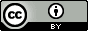## Thursday, March 17, 2016

Here are some very useful spreadsheet commands that I have been using a lot lately.  The syntax is for Google Sheets.  To use in Excel replace the ","s by ":"s

SUMIFS Returns a sum based on one or more criteria (if you only have one criteria you can use SUMIF).  Say you have

A C 2
B C 1
A D 4

You can use SUMIFS to add the "A-numbers", i.e. 6.  If you want the sum for  "A C" then use SUMIFS.

One of my side jobs is to keep track of teaching hours reported using a Google Form.  I use SUMIF to add all the reported hours together for a given person.

VLOOKUP Searches down the first column of a range for a key and returns the value of a specified cell in the row found.

You can use VLOOKUP to find the value corresponding to "B", i.e. 1.  If you use the same command to find the A value it will return the first one it found, i.e. 2.  VLOOKUP does not support multiple criteria, but if you want to look up the value for "A and D" you can use CONCATENATE to combine the two cells and lookup the value for "AD".

A more concrete example: let's say you have a long list of conformer names and energies.  You can use MIN to find the lowest energy and combine it with VLOOKUP to give you the conformer name.

CONTATENATE I've already mentioned this command, but here's another example.  In some of the papers I make tables with RMSD and $r$ values in the same column, e.g.: 0.2 (0.92).  This is easy to make from separate tables with RMSD and $r$ values with  CONCATENATE(TEXT(I4,"0.0")," (",W4,")")

SPLIT is the opposite of CONTATENATE.  SPLIT can be combined with INDEX to do some pretty nifty things.  For example INDEX(SPLIT(INDEX(SPLIT(B5,"("),0,2),"%"),0,1) will extract the number 92 from the text "B8FX10 Buried chi1: 23 correct out of 25 (92%)"

Finally the IF command is incredibly useful.  IF is actually an "if-then-else" statement.  For example IF(B15>3,"the number is bigger than 3","the number is smaller than 3") returns "the number is smaller than 3" if B15 contains the number 2.

Notice that IF commands can be combined: IF(B15>3,"the number is bigger than 3",if(B15>1,"the number is smaller than 3 but bigger than 1","the number is smaller than 1"))returns "the number is smaller than 3 but bigger than 1" B15 contains the number 2.